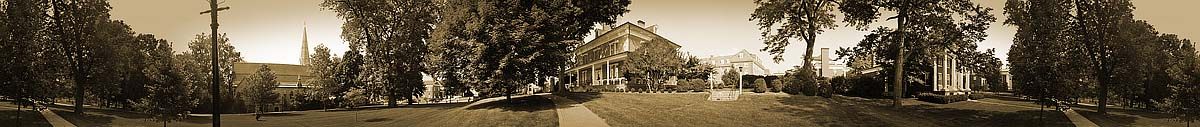R o b e r t   E .  L e e ' s   L a s t   H o m e   L e x i n g t o n
N e w  T o p o g r a p h i c s  O f  O l d  V i r g i n i a